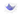← Go BackMarch 25, 20205 min read • IN tech

## Neural Net Problems - Exercise 3

Here are my solutions to exercise 3.

### Part 1

#### Question

Prove the assertion of the last paragraph. Hint: If you’re not already familiar with the Cauchy-Schwarz inequality, you may find it helpful to familiarize yourself with it.

Here is the paragraph he is referring to.

Indeed, there’s even a sense in which gradient descent is the optimal strategy for searching for a minimum. Let’s suppose that we’re trying to make a move $\Delta v$ in position so as to decrease $C$ as much as possible. This is equivalent to minimizing $\Delta C \approx \triangledown C \cdot \Delta v$. We’ll constrain the size of the move so that $\lVert \Delta v \rVert = \epsilon$ for some small fixed $\epsilon > 0$. In other words, we want a move that is a small step of a fixed size, and we’re trying to find the movement direction which decreases $C$ as much as possible. It can be proved that the choice of $\Delta v$ which minimizes $\triangledown C \cdot \Delta v$ is $\Delta v = - \eta \triangledown C$, where $\eta = \frac{\epsilon}{\lVert \triangledown C \rVert}$ is determined by the size constraint $\lVert \Delta v \rVert = \epsilon$. So gradient descent can be viewed as a way of taking small steps in the direction which does the most to immediately decrease $C$.

#### Solution

The goal here is to minimize $C$ which implies that we are trying to minimize $\Delta C$ (can be rewritten as $\Delta C = C_\text{final} - C_\text{initial}$) as best as we can. We want $C_\text{final} < C_\text{initial}$, meaning we want $\Delta C < 0$ since $\Delta C + C_\text{initial} = C_\text{final}$.

We know that $\Delta C \approx \triangledown C \cdot \Delta v$ for small fixed $\epsilon > 0$. To make $\Delta C < 0$ we must $\Delta v$ must be in a direction opposite of $\triangledown C$. This is given by the definition of a dot product. The dot product is a value that represents how much two vectors are parallel. The maximum value of a dot product is produced when two vectors are in the exact same direction (parallel) and the most negative value is produced when the two vectors are in exactly opposite directions (antiparallel). So to produce the most negative value we much choose a $\Delta v$ such that it is in the opposite direction of $\triangledown C$. We can rewrite $\Delta v = -\eta \triangledown C$. $\eta = \frac{\epsilon}{\lVert \triangledown C \rVert}$ is defined as such because we want the vector to be a fraction of the $\triangledown C$ that way we do not overshoot the minimum.### Part 2

#### Question

I explained gradient descent when $C$ is a function of two variables, and when it’s a function of more than two variables. What happens when $C$ is a function of just one variable? Can you provide a geometric interpretation of what gradient descent is doing in the one-dimensional case?

#### Solution

Please note the particular values for the graph do not matter; it is the shape of the curve that matters.

To minimize $C$ with a gradient descent algorithm is quite simple in a one-dimensional example. This is because $\triangledown C = C'(v)$ or in English the gradient is equal to the derivative of the function. So, looking at the image above, at point $v=-4$ the tangent line seems to have a negative slope and thus $\triangledown C = C'(v) < 0$, therefore when we move in the direction $- \eta \triangledown C$ we increase $v$ and move down the slope toward the right.### Part 3

#### Question

An extreme version of gradient descent is to use a mini-batch size of just $1$. That is, given a training input, $x$, we update our weights and biases according to the rules $w_k \to w_k' = w_k - \eta \frac{\partial C_x}{\partial w_k}$ and $b_l \to b_l' = b_l - \eta \frac{\partial C_x}{\partial b_l}$. Then we choose another training input, and update the weights and biases again. And so on, repeatedly. This procedure is known as online, on-line, or incremental learning. In online learning, a neural network learns from just one training input at a time (just as human beings do). Name one advantage and one disadvantage of online learning, compared to stochastic gradient descent with a mini-batch size of, say, $20$.

#### Solution

The major disadvantage of online learning is that it is much slower than stochastic gradient descent since you have to train the network over every single training input $x$. So if you have a large dataset, like the MNIST data set with 60,000 images, then online learning will have to calculate the partial derivative for each weight and biases for each image ($\frac{\partial C_x}{\partial w_k}$ and $\frac{\partial C_x}{\partial b_l}$ for a given $x$).
Stochastic gradient descent will only need to calculate the partial derivatives for a fraction of the data set (in this case, $20$ images) and then adjust the weights and biases simultaneously based on that mini-batch. This a heck of a lot faster than doing it one by one for 60,000 images!